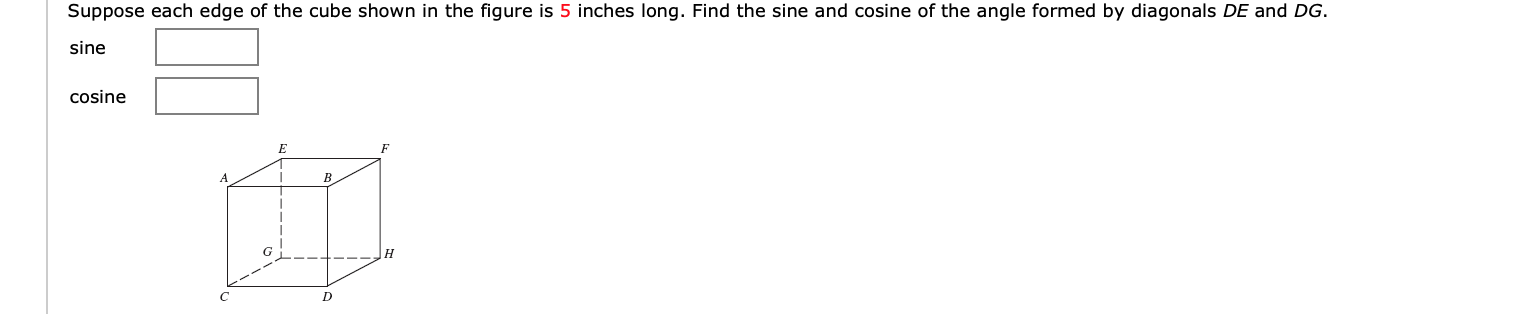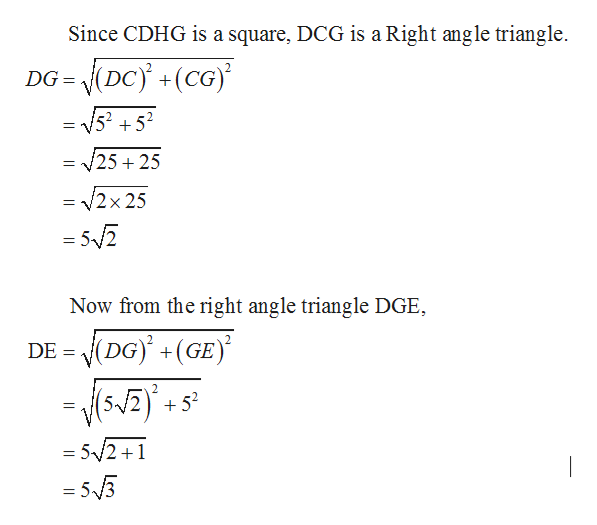# Suppose each edge of the cube shown in the figure is 5 inches long. Find the sine and cosine of the angle formed by diagonals DE and DG.sinecosineEFD

Question
12 viewshelp_outlineImage TranscriptioncloseSuppose each edge of the cube shown in the figure is 5 inches long. Find the sine and cosine of the angle formed by diagonals DE and DG. sine cosine E F D fullscreen
check_circle

Step 1

Consider the right angle triangle DGE. The length of the diagona...help_outlineImage TranscriptioncloseSince CDHG is a square, DCG is a Right angle triangle DG = /52 +52 /25 25 = 2x 25 = 52 Now from the right angle triangle DGE VDG) (GE) DE 2 52 = 5/2+1 =53 fullscreen

### Want to see the full answer?

See Solution

#### Want to see this answer and more?

Solutions are written by subject experts who are available 24/7. Questions are typically answered within 1 hour.*

See Solution
*Response times may vary by subject and question.
Tagged in

### Trigonometry